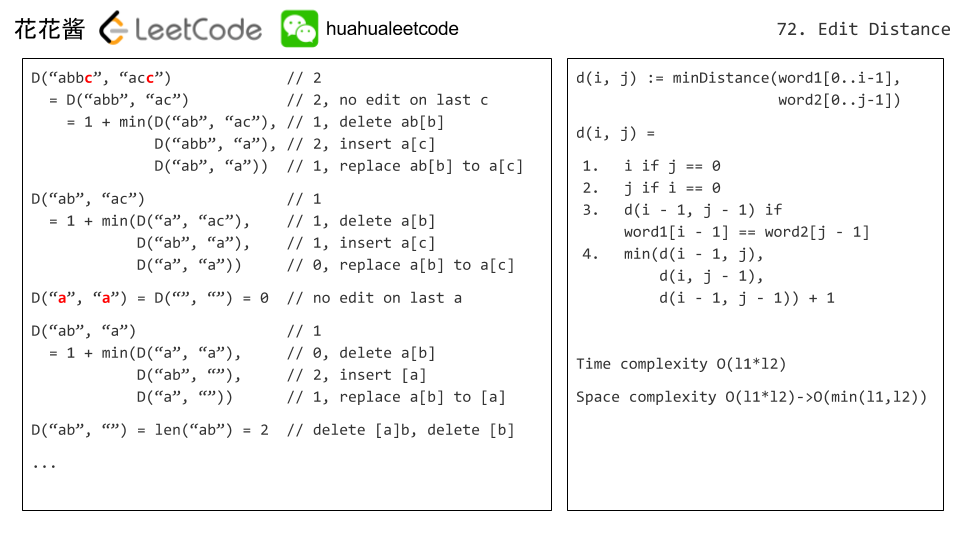Amazon

Bloomberg

Square

Uber

2020-05-31

## 72. Edit Distance

### Question:

Given two words word1 and word2, find the minimum number of operations required to convert word1 to word2.

You have the following 3 operations permitted on a word:

1. Insert a character
2. Delete a character
3. Replace a character

#### Example 1:

``````Input: word1 = "horse", word2 = "ros"
Output: 3
Explanation:
horse -> rorse (replace 'h' with 'r')
rorse -> rose (remove 'r')
rose -> ros (remove 'e')
``````

#### Example 2:

``````Input: word1 = "intention", word2 = "execution"
Output: 5
Explanation:
intention -> inention (remove 't')
inention -> enention (replace 'i' with 'e')
enention -> exention (replace 'n' with 'x')
exention -> exection (replace 'n' with 'c')
exection -> execution (insert 'u')
``````

### Solution:

Using DP to solve the questions. If character i and j are same, using `dp[i-1][j-1]`. Otherwise, there will be three cases:

1. Insert - `dp = dp + 1` For example: edit(“ab”, “ac”) = 1 + edit(“ab”, “a”) <- insert “c” at the end of word1
2. Delete - `dp = dp + 1` For example: edit(“ab”, “ac”) = 1 + edit(“a”, “ac”) <- delete “b” at the end of word1
3. Replace - `dp = dp + 1` For example: edit(“ab”, “ac”) = 1 + edit(“a”, “a”) <- replace “b” by “c” at the end of word1

The following picture demo borrowed from Huahua.``````class Solution {
public int minDistance(String word1, String word2) {
int n = word1.length();
int m = word2.length();
int[][] dp = new int[n+1][m+1];

for (int i = 0; i <= n; i++) {
dp[i]  = i;
}
for (int i = 0; i <= m; i++) {
dp[i]  = i;
}

for (int i = 1; i <= n; i++) {
for (int j = 1; j <= m; j++) {
if (word1.charAt(i - 1) == word2.charAt(j - 1)) {
dp[i][j] = dp[i-1][j-1];
} else{
dp[i][j] = 1 + Math.min(dp[i-1][j-1], Math.min(dp[i-1][j], dp[i][j-1])); // Replace, Delete, Insert
}
}
}
return dp[n][m];
}
}
``````